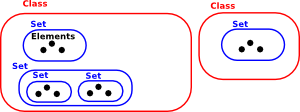# Maths - Sets - NBG

NBG is a rigorous axomatic foundation for set theory based on work by: John von Neuman, Paul Bernays and Kurt Gödel. It is one of many intended to eliminate potential paradoxes, in set theory, pointed out by Bertrand Russel (also see ZF).

 The NBG axiomatic formulation makes a distinction between 'sets' and 'classes'. All entities in NBG are classes, the word 'set' is reserved for collections that are members of other classes.So the outermost 'class' is not a 'set', this is known as a 'proper class'.

The Comprehension Principle is modified by requiring the objects referred to are sets.

### Axioms

If A and B are classes and C and D are sets:

• The axiom of Extension: Classes are equal if and only if they have the same members. For all cA, if and only if cB then A=B.
• The axiom of empty set: There exists a set C such that for all x, it is false that xC.
• The axiom of Class Comprehension: For any formula φ which does not quantify over classes, there is a class A such that xA if and only if φ(x) is true.
• The Axiom of Pairing: For any two sets C and D, there is a set with exactly C and D as members.
• The Axiom of size: For any class A, there is a set c such that c=Rep(A) if and only if there is no total one-to-one function between A and the class of all sets. This is the one that creates that definition of classes as things too big to be sets.
• The Axiom of union: for any set x, there is a set containing the members of the members of x. In other words, you can take the union of any set of sets.
• The Axiom of powerset: For any set x, there is a set which contains all of the subsets of x.
• The Axiom of infinity: There exists a set N where: * The empty set ∅ is a member of N; and * for each member x∈N, (x∪{x})∈N
• The Axiom of Foundation: All non-empty classes are disjoint from at least one of their elements.
• The Axiom of regularity: All non-empty sets are disjoint from at least one of their elements.